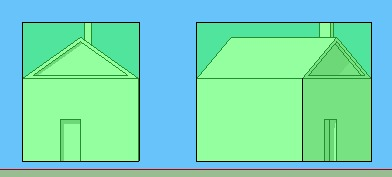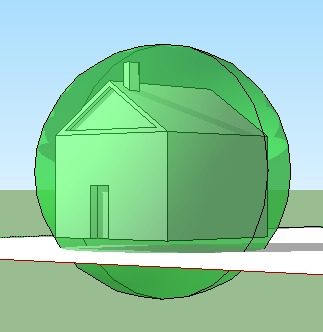To main page | 3dengine.org

# Bounding Volume

Bounding Volume of the object is a rectangular cuboid that includes all of the points of the object.The bounding volume is shown in green. Notice that for the second object (which is exactly the same), the bounding volume includes a lot of empty space, this is because often bounding volume would be calculated in either world coordinates or in camera space, instead of object-coordinates. However, this is not always so.

It is usually made of a cuboid (AABB - axis-aligned bounding box) that spans [from leftmost x coordinate to rightmost x coordinate], [from lowest y coordinate to highest y], [from nearest z to farest z]. In other words min(x) of bounding volume = min(x) of all the points of object, max(x) of volume = max(x) of all points.## Bounding spheres

The bounding spheres can also be used as bounding volume. Calculate centroid of all points (centriod.x = sum of all x's divided by number of points, centroid.y = sum of all y's divided by number of points, etc...) and radius = max(distance between center and any point - i.e. calculate distance to all points and select maximum one).## How bounding volume is used?

If cam often be used for game physics (if the bounding volumes of two object don't intersect - they don't interact physically, and it's much simpler to compute intersection of bounding volumes rather than objects themselves), occlusion culling, hidden objects calculations.